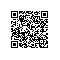# 利用辛达拉姆筛进行素数判定

## 1 常规判定方法

素数判定问题就是对给定的正整数n判定是否为素数。所谓素数。是指恰好有2个约数的整数。由于n的约数都不超过n，所以仅仅须要检查2~n-1的全部整数是否整除n就能判定是不是素数。只是，我们还能进一步优化。假设d是n的约数，那么n/d也是n的约数。由n=d*n/d可知min(d,n/d)，所以仅仅须要检查2~的全部整数就足够了。此时，素数判定的复杂度为O()。代码实现例如以下：

int classic(long long a)
{
long long half=(long long)sqrt(a);

for(int i=2;i<=half;i++)
{
if(a%i==0)  return 0;
}

return 1;
}

## 2 埃氏筛法

在介绍新方法之前。我们先回想一下埃氏筛法，这是一个与辗转相除法一样古老的算法。

大约在公元前3世纪，古希腊数学家埃拉托色尼提出了一种编造素数表的方法（如表一）。假设仅仅对一个整数进行素性判定，常规判定方法或者后面介绍的方法就足够了。

int prime[MAX_N];
bool is_prime[MAX_N];

//返回n以内的素数个数
int sieve(int n) {
int p=0;
for(int i=0;i<=n;i++) is_prime[i]=true;
is_prime=is_prime=false;
for(int i=2;i<=n;i++) {
if(is_prime[i]) {
prime[p++]=i;
for(int j=2*i;j<=n;j+=i) is_prime[j]=false;
}
}
return p;
}


## 3 辛达拉姆筛

公元1934年。一名年轻的东印度数学生辛达拉姆。提出了一种与埃拉托色尼迥然不同的筛法。它首先列出了一张表，如表二。表的第一行和第一列都是首项为4。公差为3的等差数列。从第二行開始。以后各行也是等差数列，公差分别为5,7,9,11,13……。能够看出，该表事实上是一个对称矩阵。

辛达拉姆指出：假设N出如今表中，则2N+1是合数。若N不在表中，则2N+1是素数先证明前半部分。

4+(m-1)×3=3m+1

3m+1+(n-1)*(2m+1)=2mn+m+n

再证后半部分。要想正面证明2N+1是素数是相当困难的。假设换成等价的逆否命题，即证“若2N+1不是素数，则N必在表中”似乎要easy得多。

2N+1=x*y(x ,y)为整数

则因2N+1为奇数，x、y也必为奇数。最好还是设：

x=2p+1;y=2q+1

从而2N+1=(2p+1)(2q+1)=4pq+2p+2q+1。由此能够得到

N=2pq+p+q。

综上所述。我们证明了辛达拉姆筛法的正确性。比如18不在表中，则2*18+1=37为素数。

## 4 基于辛达拉姆筛的素数推断

有了辛达拉姆筛法，我们该怎样判定一个正整数n是否为素数呢？我们仅仅须要推断(n-1)/2是否在表中就可以。

int xindalamu(long long a)
{
if(a%2==0) return 0;

long long aa=(a-1)>>1;
long long half=(long long)sqrt(aa/2);

for(int i=1;i<=half;i++)
{
if((aa-i)%(2*i+1)==0) return 0;
}

return 1;
}


我们分析一下基于辛达拉姆筛的素数判定复杂度。由for循环能够看出。复杂度也是O()。只是常系数比常规方法要小。这是由于，在求aa时有个除以二的操作，在开方时又有一个除以二的操作。所以相当于对a/4进行开方，因而最多仅仅须要对/2的行进行推断。

可是，另一个疑问须要解决，假设用户输入的是一个合数，基于辛达拉姆筛的素数判定方法是否还优于常规判定方法。能够证明，无论输入的是合数还是素数，基于辛达拉姆筛的素数判定方法始终比常规方法快一倍。证明例如以下：

假设用户输入的是素数，在分析复杂度的时候已经证明。假设用户输入的是合数，则常规方法在遇到a的最小质因子b时返回，而辛达拉姆判定方法会在遍历到第(b-1)/2行时返回。假设a=b*y，由于a是合数，则肯定存在p行和q列使得2pq+p+q=(a-1)/2。变换得(2p+1)(2q+1)=a。由于辛达拉姆判定方法在遍历时是从第一行開始的，所以我们能够觉得b=2p+1，从而辛达拉姆判定方法在第(b-1)/2行时返回。这也就证明了当输入是合数（奇数）时，基于辛达拉姆筛的素数判定方法也比常规方法快一倍。

## 5 基于辛达拉姆筛的素数筛选

辛达拉姆筛最初的目的和埃拉托色尼筛一样，也是筛选全部的素数，上面的素数判定算是一个变形应用，筛选全部素数才是筛的最重要目的。只是和埃氏筛法相比。辛达拉姆筛却不那么easy获得全部的素数。

## 參考

对于辛达拉姆筛的了解是从高中一本数学习题集中获得的，当时读了练习后面的拓展阅读，印象很深刻。以至于到如今我都留着那本习题集，感谢它。使用钉钉扫一扫加入圈子
+ 订阅